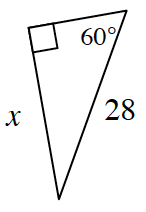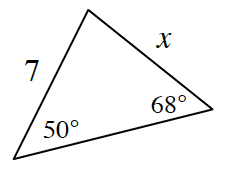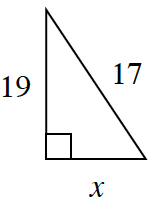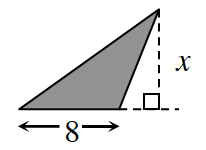### Home > CCG > Chapter Ch8 > Lesson 8.3.1 > Problem8-94

8-94.

For each triangle below, find the value of $x$, if possible. Name the triangle tool that you used. If the triangle cannot exist, explain why.

1.What special kind of triangle is this? How can this help you find $x$?

$x=14\sqrt{3}$

1.Use the Law of Sines to solve for $x$.

1.Use the Pythagorean Theorem to find $x$.

There is no solution, because the hypotenuse must be the longest side.

1.Area of the shaded region is $96$ square units.

What is the formula for the area of a triangle?